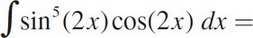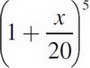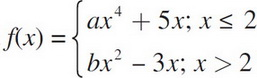# AP Calculus AB Practice Test 10

### Test Information7 questions21 minutes

Calculator Allowed

1. Find two non-negative numbers x and y whose sum is 100 and for which x2y is a maximum.

2. Find the distance traveled (to three decimal places) from t = 1 to t = 5 seconds, for a particle whose velocity is given by v(t) = t + ln t.

3.4. The volume of a cube is increasing at a rate proportional to its volume at any time t. If the volume is 8 ft3 originally, and 12 ft3 after 5 seconds, what is its volume at t = 12 seconds?

5. If f(x) =, find f″(40).

6. A particle's height at a time t ≥ 0 is given by h(t) = 100t - 16t2. What is its maximum height?

7. If f(x) is continuous and differentiable and, then b =